Reach Us+44-7482-875032
Three Dimensional Steady State Thermal Stress Analyses in a Clamped Solid Sphere | OMICS International
Journal of Applied & Computational Mathematics
All submissions of the EM system will be redirected to Online Manuscript Submission System. Authors are requested to submit articles directly to Online Manuscript Submission System of respective journal.

# Three Dimensional Steady State Thermal Stress Analyses in a Clamped Solid Sphere

Asha Bhave1*, Deshmukh KC1 and Kulkarni VS2

1Research Scholar, Department of Mathematics, R.T.M. Nagpur University, Nagpur-440010, Maharashtra, India

2Department of Mathematics, University of Mumbai, Mumbai-400098, Maharashtra India

*Corresponding Author:
Asha Bhave
Research Scholar, Department of Mathematics
RTM Nagpur University, Nagpur-440010
Maharashtra, India
Tel: 0712 253 3452
E-mail: [email protected]

Received Date: December 14, 2014; Accepted Date: February 12, 2015; Published Date: February 20, 2015

Citation: Bhave A, Deshmukh KC, Kulkarni VS (2015) Three Dimensional Steady State Thermal Stress Analyses in a Clamped Solid Sphere. J Appl Computat Math 4:205. doi: 10.4172/2168-9679.1000205

Copyright: © 2015 Bhave A, et al. This is an open-access article distributed under the terms of the Creative Commons Attribution License, which permits unrestricted use, distribution, and reproduction in any medium, provided the original author and source are credited.

Visit for more related articles at Journal of Applied & Computational Mathematics

#### Keywords

Solid sphere; Heat transfer analysis; Steady-state; Thermal stress analysis

#### Introduction

Thermoelasticity theories that admit finite speeds for thermal signals have aroused much interest in the last three decades. Spherically thermoelastic isotropy is a special case of orthogonal anisotropy in the framework of a spherical polar coordinate system and such materials have their physical properties transversely isotropic about the radius vector. For such spherically isotropic materials, a few theoretical treatments have been accomplished. The problem of thermoelastic field has been discussed by Nowinski et al. , however the temperature field is not considered. The problems of a solid sphere and a spherical cavity in an infinite solid are treated  under the assumption that the constant temperature is suddenly applied on the surface. Cheung et al.  has analyzed the transient thermal stresses in a solid sphere (Figure 1). Their assumptions include the isotropic homogeneous material and a local surface heating which results in a non-uniform temperature distribution  obtained the thermal stress distribution and the residual stresses in a spherical body where the time dependent temperature distribution is symmetrical with respect to the center of the sphere. Tanigawa et al.  considered the transient thermal stress problems of a spherical region under the condition that continuum medium possesses spherically isotropic thermoelastic property (Figure 2). Chandrasekharaiah et al. used the linear theory of thermoelasticity without energy dissipation to study waves emanating from the boundary of a spherical cavity in a homogeneous and isotropic unbounded thermoelastic body. Yapici has analyzed transient temperature and thermally-induced stress distributions in a hollow steel sphere heated by a moving uniform heat source applied on a certain zenithal segment (the heated zenithal segment), of its outer surface (the processed surface) under stagnant ambient conditions numerically (Figure 3).

In the present paper, we consider the three dimensional axisymmetric problem in a spherical coordinate system. A solid sphere is subjected to heat flux on its plane boundary surface. Heat is generated within the sphere. The attempt made to analyze heat transfer and thermal stresses of solid hemisphere under steady temperature field. The Legendre”s transforms are used for the solution of governing heat conduction equation. The solution of Navier”s equation in terms of Goodier”s thermoelastic displacement potential and the Boussinesq”s harmonic function for spherical co-ordinate system have been used to determine displacement and thermal stresses within solid. The results for temperature change, displacement and stresses have been computed numerically and illustrated graphically (Figure 4).

#### Formulation of the Problem

Following Noda et al. , Navier’s equation of thermoelasticity for axisymmetric problems may be expressed as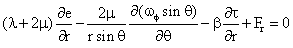(1)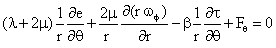(2)

Where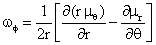The solution of the Navier’s equation without the body force for axisymmetric problems in the spherical coordinate system can be expressed [7-9], for example, by the Goodier’s thermoelastic displacement potential f and the Boussinesq harmonic functions ϕ and Ψ (Figure 5).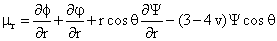(3)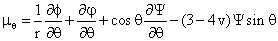(4)

Where the three functions must satisfy the equations (Figure 6)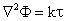(5)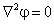(6)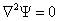(7)

Where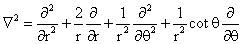(8)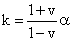(9)

Where

K is restraint coefficient.

ν is poison’s ratio.

α is thermal conductivity.

The stress components in the spherical coordinate system are represented in terms of three functions φ,ϕ and Ψ are (Figure 7)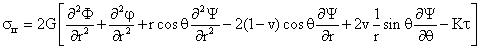(10)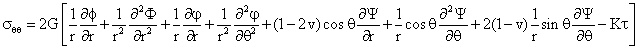(11)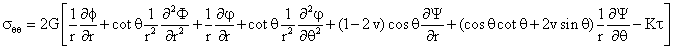(12)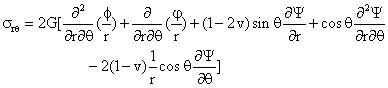(13)

The mechanical boundary conditions on the traction free surface r=b are (Figure 8)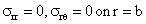(14)

The equations (1) to (14) constitute thermoelastic formulation of the problem for spherical coordinate system.

#### Governing Equation of Heat Conduction

Consider a sphere of radius b defined by 0 ≤ r ≤ b, 0 ≤ μ ≤1,0 ≤ f ≤ 2p which is subjected to arbitrary heat flux on its plane boundary surface r=b. Heat is generated within the sphere. Assume the boundary surface of a sphere is free from traction. Under these prescribed conditions, the thermal stresses are required to be determined.

A steady state temperature of a sphere satisfies the heat conduction equation (Figure 9),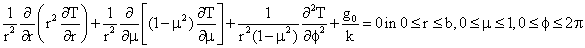(15)

subject to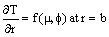(16)

where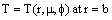(17)

#### Solution

The solution for temperature change

The Poisson’s equation (15) can be reduced to Laplace equation by defining a new dependent variable θ(r,μ,φ) as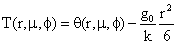(18)

Substituting equation (18) into equation (15) and (16), one obtains the Laplace equation with one inhomogeneous boundary condition (Figure 10)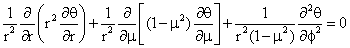(19)

With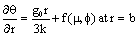(20)

The partial derivative with respect to the φ variable can be removed from the differential equation with Fourier transform. Since the range of independent variable φ is from 0 to 2 π and the temperature function is cyclic with period of 2 π, we define the Fourier transform and the corresponding inversion formula with respect to the φ variable of the temperature function (r, μ, φ ) as (Figure 11)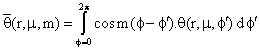(21)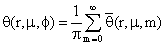(22)

By applying Integral transform defined in the equation (21) to governing heat conduction equation (15) along with the boundary condition as defined in equation (16), one obtain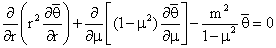in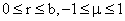(23)

With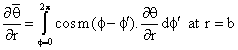(24)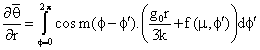(25)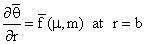(26)

The partial derivative with respect to space variable μ can be removed by means of Legendre transform. Since the range of the space variable is -1≤ μ ≤1, define Legendre transform and the corresponding inversion formula for the temperature function T (r, μ, m) with respect to μ variable as (Figure 12).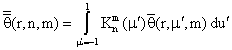(27)

And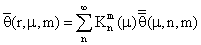(28)

where the kernel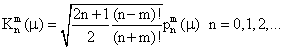(29)

By applying Legendre transform defined in the equation (27) to governing heat conduction equation (23) along with the boundary condition as defined in equation (26), one obtain (Figure 13).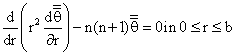(30)

With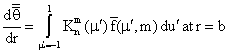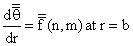(31)

on solving above equation (30), one obtain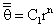(32)

using boundary condition prescribed in equation (31), one obtain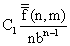(33)

hence equation (32) gives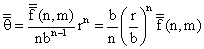(34)

hence equation (28) gives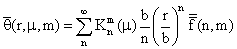(35)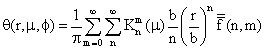(36)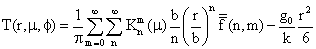(37)

using equation (37), under steady state temperature condition

t=T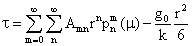(38)

Where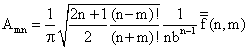(38)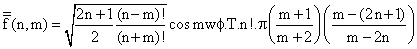(39)

The goodiers thermoelastic Displacement Potential Φ

The Goodier’s thermoelastic displacement Φ potential satisfying the equation (Figure 14).(40)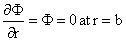(41)

using value of temperature difference from equation (38), one obtain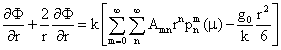(42)

on solving, one obtains the Goodier’s thermoelastic displacement potential Φ as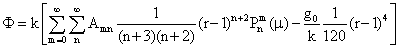(43)

The first component of displacement and thermal stresses

The first component of displacement and thermal stress with respect to Goodiers thermoelastic displacement potential function Ф can be obtained as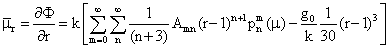(44)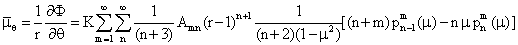(45)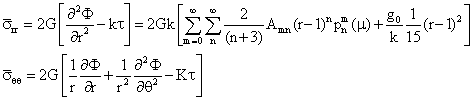(46)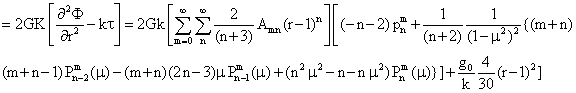(47)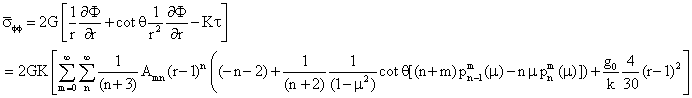(48)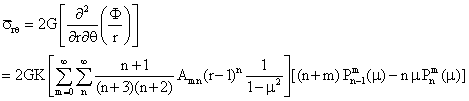(49)

The solution of laplace equation

The solution of Laplace equation satisfying conditions given in equation (6) and (7) are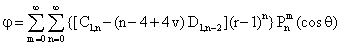(50)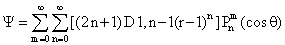(51)

The second component of displacement and thermal stresses

The displacements and stresses due to displacement function φ and Ѱ are (Figure 15)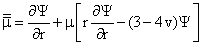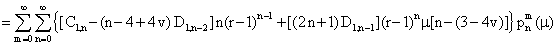(52)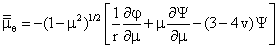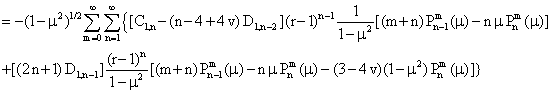(53)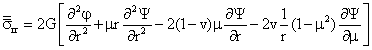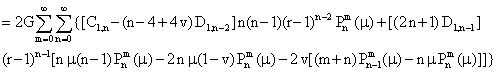(54)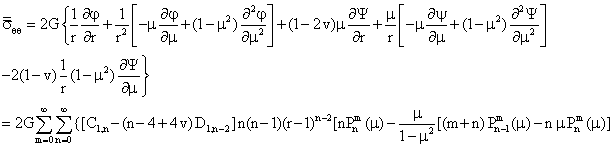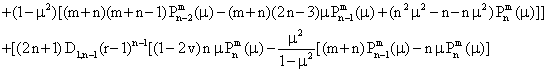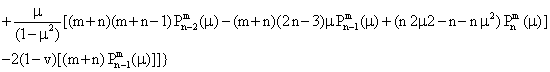(55)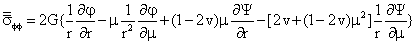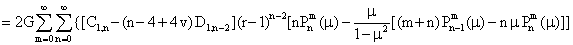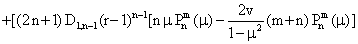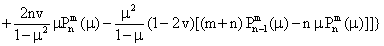(56)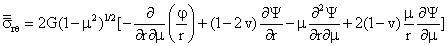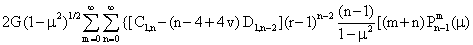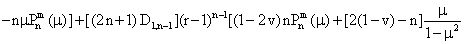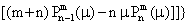(57)

The displacement and thermal stresses

The displacements and thermal stresses defined in equations (3), (4) and (10) to (14) can be obtain by adding (44) to (49) and (52) to (57) respectively as (Figure 16)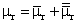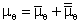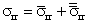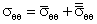The unknowns used in equations (49) and (50), can be determined by solving equilibrium equation (14) as

C1,n = 0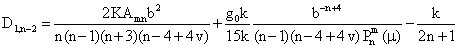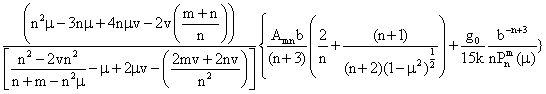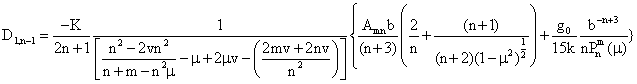#### Numerical Calculations

Special case

Setting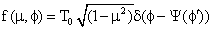Heat transfer coefficient h=10

Strengthening of heat flux 0 T=100°C

Material properties

Thermal diffusivity a 20.34×10-6 (m2s-1),

Thermal Conductivity k=72.7(W/mK)

Lame constant μ=0.5,

Poisson ration=0.35,

Heat transfer coefficient h=10

Strengthening of heat flux 0 T=100°C

Dimensions

Radius of Sphere b=1m,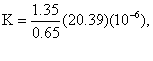G=0.5

#### Concluding Remarks

In this paper a clamped solid sphere is considered and determined the expressions for temperature, displacement and thermal stresses at different values of μ and f. As a special case mathematical model is constructed for iron (pure) solid sphere subjected to heat flux at the spherical boundary with the material properties specified as above.

In order to study the characteristic of temperature variation, displacement and stresses within solid sphere we have performed numerical calculation for special case and illustrated these variations graphically along radial direction.

Due to internal heat generation and heat flux applied on spherical boundary surface, heat flow can be observed towards the outer spherical boundary of the sphere from center of sphere. At the same Time radial and angular displacements seen at the center. It means solid displacement and heat flow are opposite to each other.

The tensile thermal stresses can be observed around center of sphere where as in the rotation of sphere tensile as well compressive thermal stresses are seen. All stresses are negligible towards the outer spherical boundary of the sphere.

It may be concluded that, clamped spherical boundary restrict expansion at outer surface hence displacement and thermal stresses developed around center which may results in vertical expansion of solid sphere.

#### References

Select your language of interest to view the total content in your interested language

### Article Usage

• Total views: 12200
• [From(publication date):
February-2015 - Aug 24, 2019]
• Breakdown by view type
• HTML page views : 8389
• PDF downloads : 3811

## Post your commentCan't read the image? click here to refresh
###### Peer Reviewed Journals

Make the best use of Scientific Research and information from our 700 + peer reviewed, Open Access Journals

International Conferences 2019-20

Meet Inspiring Speakers and Experts at our 3000+ Global Annual Meetings

Top